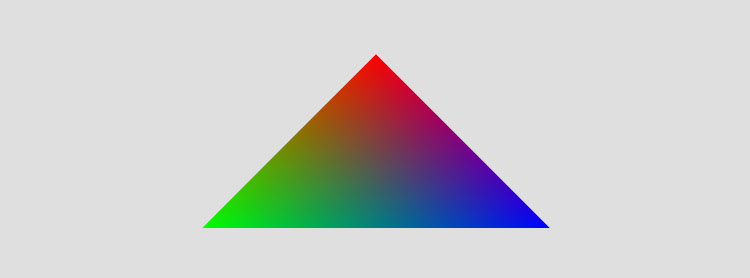# Vertex Colors in Three.jsVertex colors in three.js can be easily achieved using the built in geometry.vertices.push function and the THREE.VertexColors attribute when setting up a custom GLSL shader:

First, draw the geometry:

``````var geometry = new THREE.Geometry();
geometry.vertices.push(
new THREE.Vector3(0, 0, 0),
new THREE.Vector3(-2, -2, 0),
new THREE.Vector3(2, -2, 0));

geometry.faces.push(new THREE.Face3(0, 1, 2));

geometry.faces.vertexColors = new THREE.Color("rgb(255,0,0)");
geometry.faces.vertexColors = new THREE.Color("rgb(0,255,0)");
geometry.faces.vertexColors = new THREE.Color("rgb(0,0,255)");
``````

This makes three points facing the camera (distance may vary depending on how your camera is set up). Then assigns a color to each one using THREE.Color

Next, create a custom shader ( if you need to know more about custom shaders basics see Basic GLSL Displacement Shader in Three.js? ) and load it with some attributes before adding the geometry to the scene:

``````var shader = THREE.VertexColorShader;

var parameters = {
//this activates the 'colors' attribute (uses vertex colors stored in the created geometry)
vertexColors: THREE.VertexColors,
side: THREE.DoubleSide,
uniforms: uniforms};

mesh = new THREE.Mesh(geometry, material);
mesh.position.y = 1;
``````

note the vertexColors parameter. This is built into three.js and specifies how the vertices will be rendered. If we set it to THREE.VertexColors then we can use the “color” attribute in the GLSL shader to access the colors already assigned to the vertices.

``````THREE.VertexColorShader = {

uniforms: {
},
"varying vec3 vColor;",
"void main() {",
"vColor = color;",
"gl_Position = projectionMatrix * modelViewMatrix * vec4( position, 1.0 );",
"}"
].join("\n"),
"varying vec3 vColor;",
"void main( void ) {",
"gl_FragColor = vec4( vColor.rgb, 1. );",
"}"
].join("\n")
};
``````

As mentioned, the color attribute is used. We copy it into vColor, then pass it to the fragment shader to blend?(interpolate) it across each vertex.